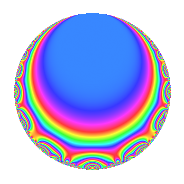# Properties

 Label 2003.1.b.bLevel 2003 Weight 1 Character orbit 2003.b Self dual Yes Analytic conductor 1.000 Analytic rank 0 Dimension 3 Projective image $$D_{9}$$ CM disc. -2003 Inner twists 2

# Related objects

## Newspace parameters

 Level: $$N$$ = $$2003$$ Weight: $$k$$ = $$1$$ Character orbit: $$[\chi]$$ = 2003.b (of order $$2$$ and degree $$1$$)

## Newform invariants

 Self dual: Yes Analytic conductor: $$0.999627220304$$ Analytic rank: $$0$$ Dimension: $$3$$ Coefficient field: $$\Q(\zeta_{18})^+$$ Coefficient ring: $$\Z[a_1, a_2, a_3]$$ Coefficient ring index: $$1$$ Projective image $$D_{9}$$ Projective field Galois closure of 9.1.16096216216081.1 Artin image size $$18$$ Artin image $D_9$ Artin field Galois closure of 9.1.16096216216081.1

## $q$-expansion

Coefficients of the $$q$$-expansion are expressed in terms of a basis $$1,\beta_1,\beta_2$$ for the coefficient ring described below. We also show the integral $$q$$-expansion of the trace form.

 $$f(q)$$ $$=$$ $$q -\beta_{1} q^{3} + q^{4} + ( 1 + \beta_{2} ) q^{9} +O(q^{10})$$ $$q -\beta_{1} q^{3} + q^{4} + ( 1 + \beta_{2} ) q^{9} -\beta_{1} q^{12} + ( \beta_{1} - \beta_{2} ) q^{13} + q^{16} - q^{19} + q^{25} + ( -1 - \beta_{1} ) q^{27} + ( 1 + \beta_{2} ) q^{36} + ( -1 + \beta_{1} - \beta_{2} ) q^{39} + ( \beta_{1} - \beta_{2} ) q^{47} -\beta_{1} q^{48} + q^{49} + ( \beta_{1} - \beta_{2} ) q^{52} - q^{53} + \beta_{1} q^{57} + \beta_{2} q^{59} + q^{64} + \beta_{2} q^{73} -\beta_{1} q^{75} - q^{76} + \beta_{2} q^{79} + ( 1 + \beta_{1} ) q^{81} - q^{89} +O(q^{100})$$ $$\operatorname{Tr}(f)(q)$$ $$=$$ $$3q + 3q^{4} + 3q^{9} + O(q^{10})$$ $$3q + 3q^{4} + 3q^{9} + 3q^{16} - 3q^{19} + 3q^{25} - 3q^{27} + 3q^{36} - 3q^{39} + 3q^{49} - 3q^{53} + 3q^{64} - 3q^{76} + 3q^{81} - 3q^{89} + O(q^{100})$$

Basis of coefficient ring in terms of $$\nu = \zeta_{18} + \zeta_{18}^{-1}$$:

 $$\beta_{0}$$ $$=$$ $$1$$ $$\beta_{1}$$ $$=$$ $$\nu$$ $$\beta_{2}$$ $$=$$ $$\nu^{2} - 2$$
 $$1$$ $$=$$ $$\beta_0$$ $$\nu$$ $$=$$ $$\beta_{1}$$ $$\nu^{2}$$ $$=$$ $$\beta_{2} + 2$$

## Character Values

We give the values of $$\chi$$ on generators for $$\left(\mathbb{Z}/2003\mathbb{Z}\right)^\times$$.

 $$n$$ $$5$$ $$\chi(n)$$ $$-1$$

## Embeddings

For each embedding $$\iota_m$$ of the coefficient field, the values $$\iota_m(a_n)$$ are shown below.

For more information on an embedded modular form you can click on its label.

Label $$\iota_m(\nu)$$ $$a_{2}$$ $$a_{3}$$ $$a_{4}$$ $$a_{5}$$ $$a_{6}$$ $$a_{7}$$ $$a_{8}$$ $$a_{9}$$ $$a_{10}$$
2002.1
 1.87939 −0.347296 −1.53209
0 −1.87939 1.00000 0 0 0 0 2.53209 0
2002.2 0 0.347296 1.00000 0 0 0 0 −0.879385 0
2002.3 0 1.53209 1.00000 0 0 0 0 1.34730 0
 $$n$$: e.g. 2-40 or 990-1000 Significant digits: Format: Complex embeddings Normalized embeddings Satake parameters Satake angles

## Inner twists

Char. orbit Parity Mult. Self Twist Proved
1.a Even 1 trivial yes
2003.b Odd 1 CM by $$\Q(\sqrt{-2003})$$ yes

## Hecke kernels

This newform can be constructed as the kernel of the linear operator $$T_{3}^{3} - 3 T_{3} + 1$$ acting on $$S_{1}^{\mathrm{new}}(2003, [\chi])$$.